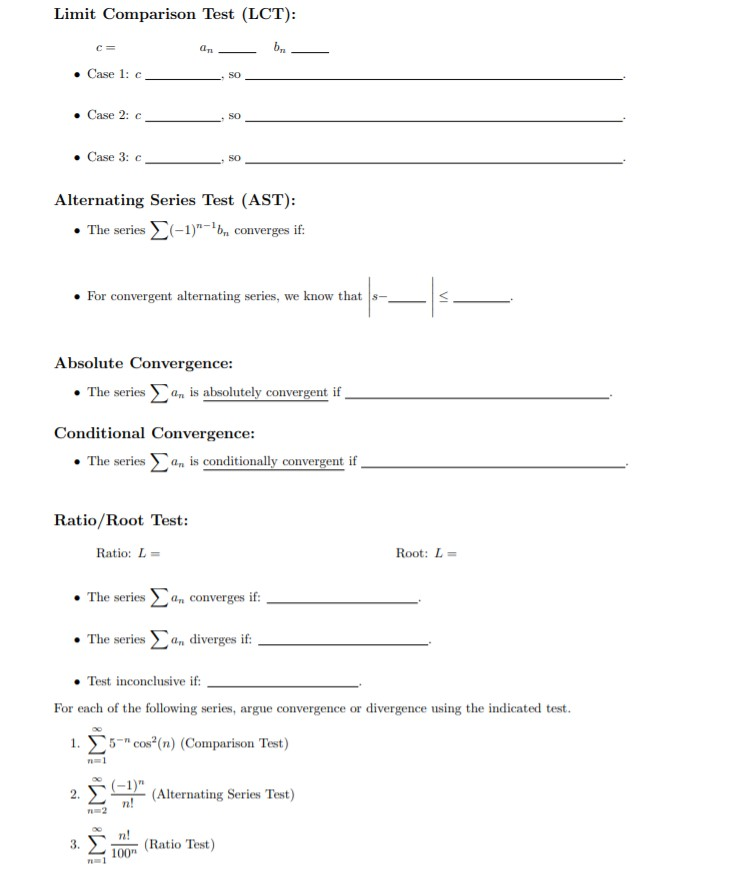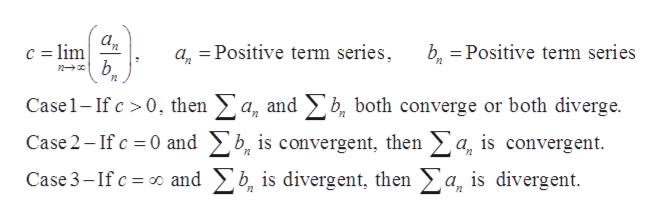# Limit Comparison Test (LCT):c=Case 1: cSOCase 2: cSOCase 3: cSOAlternating Series Test (AST):The series-1)bn converges if:For convergent alternating series, we know that s-,Absolute Convergence:The seriesan is absolutely convergent ifConditional Convergence:The seriesan is conditionally comvergent ifRatio/Root Test:Ratio: LRoot: L=The seriesan converges if:The seriesan diverges if:Test inconclusive if:For each of the following series, argue convergence or divergence using the indicated test.1. 5Cos(n) (Comparison Test)(1)(Alternating Series Test)n!2.2n!(Ratio Test)1003.VI

Question
2 views

For each of the following, fill in the blanks to complete the description of the test.help_outlineImage TranscriptioncloseLimit Comparison Test (LCT): c= Case 1: c SO Case 2: c SO Case 3: c SO Alternating Series Test (AST): The series -1)bn converges if: For convergent alternating series, we know that s-, Absolute Convergence: The seriesan is absolutely convergent if Conditional Convergence: The seriesan is conditionally comvergent if Ratio/Root Test: Ratio: L Root: L= The seriesan converges if: The seriesan diverges if: Test inconclusive if: For each of the following series, argue convergence or divergence using the indicated test. 1. 5Cos(n) (Comparison Test) (1)(Alternating Series Test) n! 2. 2 n! (Ratio Test) 100 3. VI fullscreen
check_circle

Step 1

Since we only answer up to 3 sub-parts, we’ll answer the first 3.

Please resubmit the question and specify the other subparts (up to 3) you’d like answered.

Step 2

Limit Comparison test-

Assume,help_outlineImage Transcriptionclosec lim n baPositive term series a Positive term series 2 Case1-If c 0, then a, and b both converge or both diverge. Case 2-If c 0 and b, is convergent, then >a, is convergent Case 3-If c and b, is divergent, then a, is divergent fullscreen
Step 3

Alternating series test-

1)The ...

### Want to see the full answer?

See Solution

#### Want to see this answer and more?

Solutions are written by subject experts who are available 24/7. Questions are typically answered within 1 hour.*

See Solution
*Response times may vary by subject and question.
Tagged in

### Math